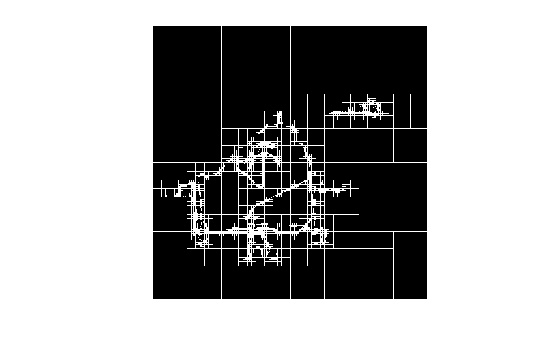# qtdecomp

## Syntax

``S = qtdecomp(I)``
``S = qtdecomp(I,threshold)``
``S = qtdecomp(I,threshold,mindim)``
``````S = qtdecomp(I,threshold,[mindim maxdim])``````
``S = qtdecomp(I,fun)``

## Description

````S = qtdecomp(I)` performs a quadtree decomposition on the grayscale image `I` and returns the quadtree structure in the sparse matrix `S`. By default, `qtdecomp` splits a block unless all elements in the block are equal.```

example

````S = qtdecomp(I,threshold)` splits a block if the maximum value of the block elements minus the minimum value of the block elements is greater than `threshold`.```
````S = qtdecomp(I,threshold,mindim)` will not produce blocks smaller than `mindim`, even if the resulting blocks do not meet the threshold condition.```
``````S = qtdecomp(I,threshold,[mindim maxdim])``` will not produce blocks smaller than `mindim` or larger than `maxdim`. Blocks larger than `maxdim` are split even if they meet the threshold condition.```
````S = qtdecomp(I,fun)` uses the function `fun` to determine whether to split a block.```

## Examples

collapse all

Create a small sample matrix.

```I = uint8([1 1 1 1 2 3 6 6;... 1 1 2 1 4 5 6 8;... 1 1 1 1 7 7 7 7;... 1 1 1 1 6 6 5 5;... 20 22 20 22 1 2 3 4;... 20 22 22 20 5 4 7 8;... 20 22 20 20 9 12 40 12;... 20 22 20 20 13 14 15 16]);```

Perform the quadtree decomposition and display the results.

```S = qtdecomp(I,.05); disp(full(S));```
``` 4 0 0 0 4 0 0 0 0 0 0 0 0 0 0 0 0 0 0 0 0 0 0 0 0 0 0 0 0 0 0 0 4 0 0 0 2 0 2 0 0 0 0 0 0 0 0 0 0 0 0 0 2 0 1 1 0 0 0 0 0 0 1 1 ```

`I = imread('liftingbody.png');`

Perform the quadtree decomposition and display the block representation in a figure.

```S = qtdecomp(I,.27); blocks = repmat(uint8(0),size(S)); for dim = [512 256 128 64 32 16 8 4 2 1]; numblocks = length(find(S==dim)); if (numblocks > 0) values = repmat(uint8(1),[dim dim numblocks]); values(2:dim,2:dim,:) = 0; blocks = qtsetblk(blocks,S,dim,values); end end blocks(end,1:end) = 1; blocks(1:end,end) = 1; imshow(I)``````figure imshow(blocks,[])```## Input Arguments

collapse all

Grayscale image, specified as an m-by-n numeric matrix. If the syntax includes a function handle, `fun`, then the image can be of any class supported by the function.

Data Types: `single` | `double` | `int16` | `uint8` | `uint16` | `logical`

Threshold of block homogeneity, specified as a scalar in the range [0, 1].

• If `I` is of class `uint8`, then `qtdecomp` multiplies the value of `threshold` by 255 to determine the actual threshold to use.

• If `I` is of class `uint8`, then `qtdecomp` multiplies the value of `threshold` by 65535 to determine the actual threshold to use.

Minimum block size, specified as a positive integer. `mindim` must be a factor of the image size.

Maximum block size, specified as a positive integer. `maxdim/mindim` must be a power of 2.

Function handle, specified as a handle. The function must accept as input all m-by-m blocks stacked into an m-by-m-by-k array, where k is the number of blocks. The function must return a logical k-element vector, whose values are `1` if the corresponding block should be split, and `0` otherwise. For example, if k(`3`) is `0`, then the third m-by-m block should not be split.

## Output Arguments

collapse all

Quadtree structure, returned as a sparse matrix. If `S(k,m)` is nonzero, then `(k,m)` is the upper left corner of a block in the decomposition, and the size of the block is given by `S(k,m)`.

Data Types: `double`

## Tips

• `qtdecomp` is appropriate primarily for square images whose dimensions are a power of 2, such as 128-by-128 or 512-by-512. These images can be divided until the blocks are as small as 1-by-1. If you use `qtdecomp` with an image whose dimensions are not a power of 2, at some point the blocks cannot be divided further. For example, if an image is 96-by-96, it can be divided into blocks of size 48-by-48, then 24-by-24, 12-by-12, 6-by-6, and finally 3-by-3. No further division beyond 3-by-3 is possible. To process this image, you must set `mindim` to 3 (or to 3 times a power of 2); if you are using the syntax that includes a function, `fun`, the function must return `0` at the point when the block cannot be divided further.

## Algorithms

The `qtdecomp` function divides a square image into four equal-sized square blocks, and then tests each block to see if it meets some criterion of homogeneity. If a block meets the criterion, it is not divided any further. If it does not meet the criterion, it is subdivided again into four blocks, and the test criterion is applied to those blocks. This process is repeated iteratively until each block meets the criterion. The result can have blocks of several different sizes.